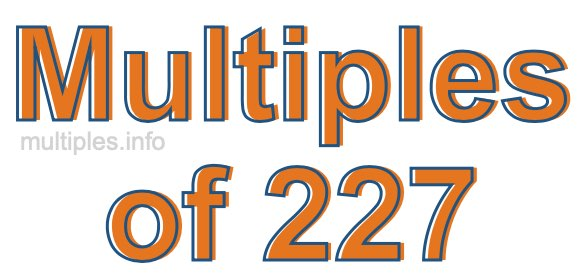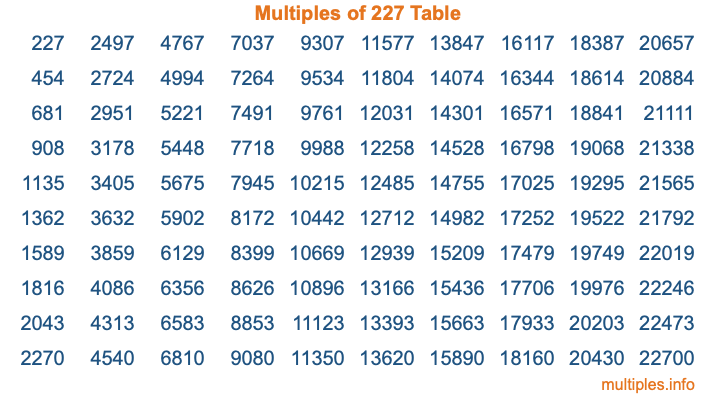Multiples of 227Welcome to the Multiples of 227 page. Here we will first teach you everything you will ever need to know about the multiples of 227, and then give you a study guide summary of everything we taught you to make sure you remember it all. Use this page to look up facts and learn information about the multiples of 227. This page will make you a multiples of two hundred twenty-seven expert!

Definition of Multiples of 227
Multiples of 227 are all the numbers that when divided by 227 equal an integer. Each of the multiples of 227 are called a multiple. A multiple of 227 is created by multiplying 227 by an integer.

Therefore, to create a list of multiples of 227, you start with 1 multiplied by 227, then 2 multiplied by 227, then 3 multiplied by 227, and so on for as long as you want. Thus, the list of the first five multiples of 227 is 227, 454, 681, 908, and 1135. To see a larger list of multiples of 227, see the printable image of Multiples of 227 further down on this page. We also have a category where you can choose any nth multiple of 227.

Multiples of 227 Checker
The Multiples of 227 Checker below checks to see if any number of your choice is a multiple of 227. In other words, it checks to see if there is any number (integer) that when multiplied by 227 will equal your number. To do that, we divide your number by 227. If the the quotient is an integer, then your number is a multiple of 227.

Is  a multiple of 227?

Least Common Multiple of 227 and ...
A Least Common Multiple (LCM) is the lowest multiple that two or more numbers have in common. This is also called the smallest common multiple or lowest common multiple and is useful to know when you are adding our subtracting fractions. Enter one or more numbers below (227 is already entered) to find the LCM.

Check out our LCM Calculator if you need more details about the Least Common Multiple or if you need the LCM for different numbers for adding and subtraction fractions.

nth Multiple of 227
As we stated above, 227 is the first multiple of 227, 454 is the second multiple of 227, 681 is the third multiple of 227, and so on. Enter a number below to find the nth multiple of 227.

th multiple of 227

Multiples of 227 vs Factors of 227
227 is a multiple of 227 and a factor of 227, but that is where the similarities end. All postive multiples of 227 are 227 or greater than 227. All positive factors of 227 are 227 or less than 227.

Below is the beginning list of multiples of 227 and the factors of 227 so you can compare:

Multiples of 227: 227, 454, 681, 908, 1135, etc.

Factors of 227: 1, 227

As you can see, the multiples of 227 are all the numbers that you can divide by 227 to get a whole number. The factors of 227, on the other hand, are all the whole numbers that you can multiply by another whole number to get 227.

It's also interesting to note that if a number (x) is a factor of 227, then 227 will also be a multiple of that number (x).

Multiples of 227 vs Divisors of 227
The divisors of 227 are all the integers that 227 can be divided by evenly. Below is a list of the divisors of 227.

Divisors of 227: 1, 227

The interesting thing to note here is that if you take any multiple of 227 and divide it by a divisor of 227, you will see that the quotient is an integer.

Multiples of 227 Table
Below is an image of the first 100 multiples of 227 in a table. The table is in chronological order, column by column. The first column has the first ten multiples of 227, the second column has the next ten multiples of 227, and so on.The Multiples of 227 Table is also referred to as the 227 Times Table or Times Table of 227. You are welcome to print out our table for your studies.

Negative Multiples of 227
Although not often discussed or needed in math, it is worth mentioning that you can make a list of negative multiples of 227 by multiplying 227 by -1, then by -2, then by -3, and so on, to get the following list of negative multiples of 227:

-227, -454, -681, -908, -1135, etc.

Multiples of 227 Summary
Below is a summary of important Multiples of 227 facts that we have discussed on this page. To retain the knowledge on this page, we recommend that you read through the summary and explain to yourself or a study partner why they hold true.

There are an infinite number of multiples of 227.

A multiple of 227 divided by 227 will equal a whole number.

227 divided by a factor of 227 equals a divisor of 227.

The nth multiple of 227 is n times 227.

The largest factor of 227 is equal to the first positive multiple of 227.

227 is a multiple of every factor of 227.

227 is a multiple of 227.

A multiple of 227 divided by a divisor of 227 equals an integer.

227 divided by a divisor of 227 equals a factor of 227.

Any integer times 227 will equal a multiple of 227.

Multiples of a Number
Here you can get the multiples of another number, all with the same attention to detail as we did for multiples of 227 on this page.

Multiples of
Multiples of 228
Did you find our page about multiples of two hundred twenty-seven educational? Do you want more knowledge? Check out the multiples of the next number on our list!